# for循环(entry-condition loop)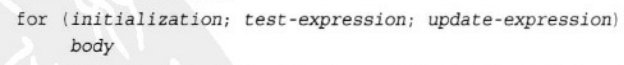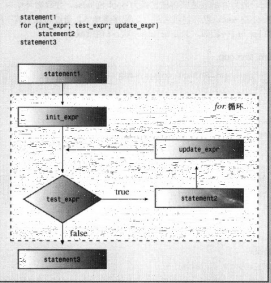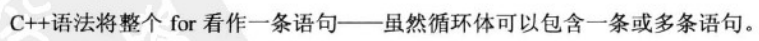#include <iostream>
int main()
{
int i;//计数器
//初始化(initialization)；测试表达式(loop test)；更新表达式(loop update)
for(i = 0; i < 5; i++)
std::cout << "C++ knows loops.\n";
std::cout << "C++ knows when to stop.\n";
return 0;
}


#include <iostream>
int main()
{
std::cout << "Enter the starting countdown limit(a positive integer):\n";
int limit;
std::cin >> limit;
int i;
for(i = limit; i; i--)
std::cout << "i = " << i << std::endl;//不写namespace就会发现，
//cin cout的前缀还好，但endl的前缀老忘！！
std::cout << "Now i = " << i << std::endl;
return 0;
}

Enter the starting countdown limit(a positive integer):
5
i = 5
i = 4
i = 3
i = 2
i = 1
Now i = 0


## 表达式（值 或 值与运算符的组合）

C++的每个表达式都有值。

x = 20是一个值为20的表达式，和C一样，C++赋值表达式的值被定义为左侧成员的值。实际上C和C++支持的多重赋值就是用了这个特性，当然也用到了赋值运算符从右往左的结合性，所以会把右边的值依次给左边，0先给z，(z = 0)的值再给y

x = y = z = 0;//z = 0的值是0，所以y = z的值是0，依次···


### 示例 表达式的值（如何打印true和false而非0和1）

#include <iostream>
int main()
{
using namespace std;
int x;

cout << (x = 100) << endl;//不打括号报错，因为插入运算符的优先级比赋值运算符更高
cout << x << endl;
cout << (x < 3) << endl;//cout显示bool之前会转换为int
cout << (x > 3) << endl;
cout.setf(ios_base::boolalpha);//新的C++特征，设置一个标记，命令cout显示true和false而不是int的0和1
cout << (x < 3) << endl;
cout << (x > 3) << endl;
return 0;
}

100
100
0
1
false
true


### 表达式语句：所有表达式加上分号就是表达式语句

x + 15;//是有效的表达式语句，但是得到的结果没存起来，后面没法用，比较智能的编译器做优化会直接忽略这种语句


### 示例 求阶乘

#include <iostream>
const int ArraySize = 16;//用const值定义数组的元素个数是个好习惯；声明外部变量，这使得ArraySize在程序运行期间一直存在，且文件内所有函数均可共用，其他问价也可以用
int main()
{
long long factorials[ArraySize] = {};//阶乘很大，增长很快，因此选择long long
factorials = factorials = 1;
for (int i = 2; i < ArraySize; i++)//i<ArraySize和i<=ArraySize-1效果一样，却更简洁
factorials[i] = i * factorials[i - 1];
for (int i = 0; i < ArraySize; i++)
std::cout << i << "! = " << factorials[i] << std::endl;
return 0;
}

0! = 1
1! = 1
2! = 2
3! = 6
4! = 24
5! = 120
6! = 720
7! = 5040
8! = 40320
9! = 362880
10! = 3628800
11! = 39916800
12! = 479001600
13! = 6227020800
14! = 87178291200
15! = 1307674368000


### 示例 步长不为1，使用using声明

#include <iostream>
int main()
{
using std::cout;//using声明
using std::cin;
using std::endl;

cout << "Enter an integer:\n";
int by;
cin >> by;
for (int i = 0; i < 100; i = i + by)
cout << "i = " << i << endl;
return 0;
}

Enter an integer:
10
i = 0
i = 10
i = 20
i = 30
i = 40
i = 50
i = 60
i = 70
i = 80
i = 90


### 示例 反序输出字符串

#include <iostream>
int main()
{
using std::cin;
using std::cout;
using std::string;

cout << "Enter a word:";
string word;
cin >> word;
for (int i = word.size() - 1; i>=0; i--)//反序计数
cout << word[i];//使用数组表示法访问string对象的每一个字符
return 0;
}

Enter a word:animal
lamina


Enter a word:redder
redder


### 示例 ++ – prefix postfix

#include <iostream>
int main()
{
using std::cout;
using std::endl;

int a, b, c, d;
a = b = 6;
cout << "a = " << a << ", b = " << b << endl;
cout << "a++ = " << a++ << ", ++b = " << ++b << endl;
cout << "a = " << a << ", b = " << b << endl;

c = ++a;
d = b++;
cout << "c = " << c << endl;
cout << "d = " << d << endl;
return 0;
}


a = 6, b = 6
a++ = 6, ++b = 7
a = 7, b = 7
c = 8
d = 7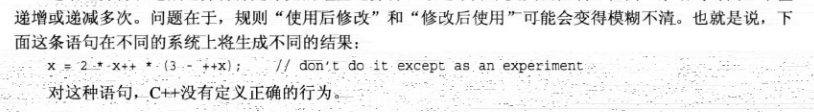#### 前缀版本效率比后缀高！

i++;
++i;

for (int i = 0; i < n; i++)
for (int i = 0; i < n; ++i)


#### *++pt, ++*pt, *pt++, (*pt)++, *(pt++)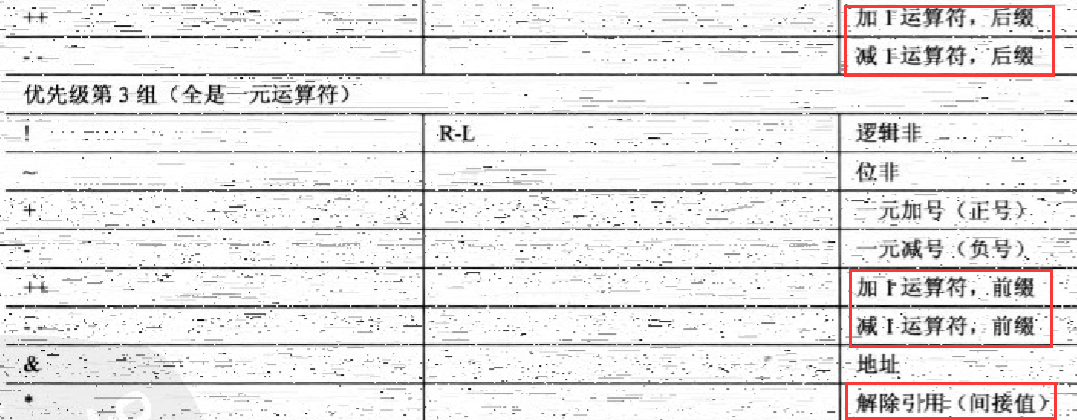int a = {1, 4, 6, 10, 5};
int *pt = a;


∗ * ++pt:

++ ∗ * pt:
pt先和解引用结合，所以递增的是1，结果是2

∗ * pt++:

*pt;
pt++;


∗ * (pt++),这个和上一个的区别就是解引用的是pt递增后的值，最终结果是4，pt也指向4

∗ * pt）++:这个就简单了，就是解引用以后的值递增，等于2

## 逗号运算符（优先级最低的运算符），把多个表达式合并为一个

i = 20, j = 2 * i;//j等于40；整个表达式的值是40
cats = (17, 23);//cats = 23
cats = 17, 23;//cats = 17
(cats = 17), 23;//cats = 17,和上一句等价，因为逗号运算符优先级是最低的


### 示例 字符串反转（反序输出）

#include <iostream>
int main()
{
using std::cout;
using std::cin;
using std::string;

cout << "Enter a word:\n";
string word;
cin >> word;

char temp;
int i, j;//必须在循环外部声明
for (i = word.size() - 1, j = 0; j < i; ++j, --i)
//用了两次逗号运算符，这里需要注意的是：
//绝对不可以用逗号运算符组合两个声明，因为那不是表达式！！
{//交换第一个和最后一个字符；第二个和倒数第二个字符···直到相遇
temp = word[j];
word[j] = word[i];
word[i] = temp;
}
cout << word << "\nDone!\n";
return 0;
}

Enter a word:
lobster
retsbol
Done!


## 关系表达式（优先级低于算术运算符）

C++有6种关系运算符。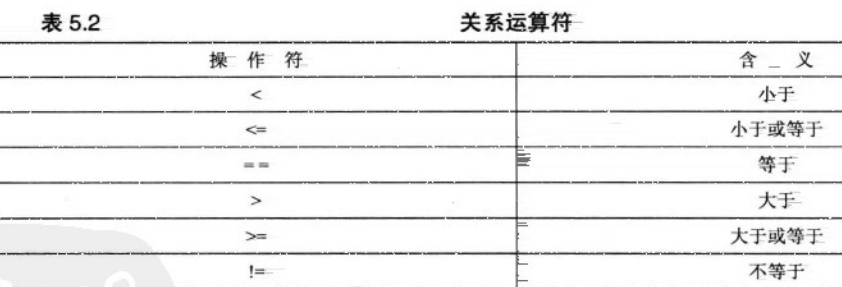x + 3 > y - 2;//等价于(x + 3) > (y -  2)
x + (3 > y) - 2;//也是有效表达式，因为bool会被提升为int


### 关系运算符不能比较字符串，用strcmp()

#### 示例 比较字符

#include <iostream>
int main()
{
using std::cout;
for (char ch = 'a'; ch < 'f'; ++ch)
cout << ch << '\n';
return 0;
}

a
b
c
d
e


C里面说过，strcmp()函数接收两个地址，所以可以是char数组名，指针，字符串字面量。

#### 示例 比较字符串 strcmp()的返回值作为for循环测试条件(因吹斯听）（非计数循环)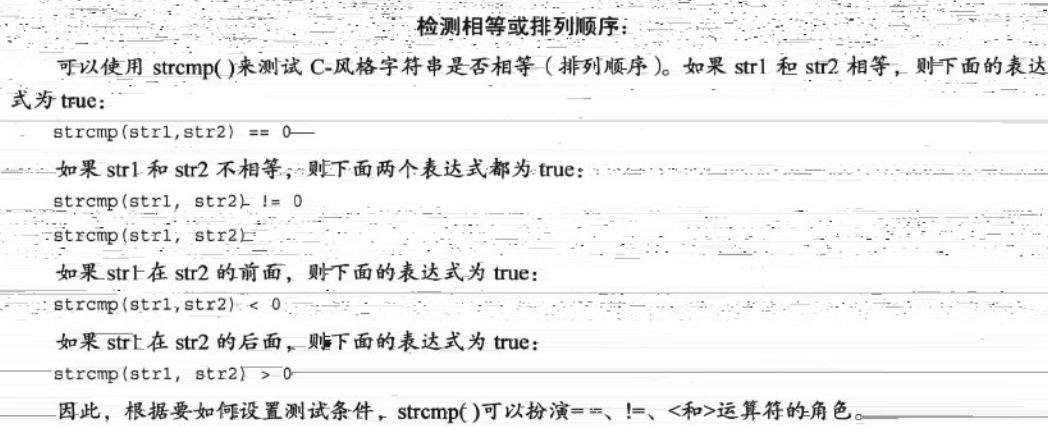#include <iostream>
#include <cstring>
int main()
{
using namespace std;
char word = "?ate";

for (char ch = 'a'; strcmp(word, "mate"); ++ch)//测试条件等价于strcmp(word, "mate")!=0
{
cout << word << endl;
word = ch;
}
cout << "After loops, word = " << word << endl;
return 0;
}

?ate
aate
bate
cate
date
eate
fate
gate
hate
iate
jate
kate
late
After loops, word = mate


#### 示例 用关系运算符比较string类字符串对象（运算符重载）（非计数循环）

string类的对象之所以又可以用关系运算符比较字符串，是因为string类重载了关系运算符，即重新定义了这些关系运算符

#include <iostream>
#include <string>
int main()
{
using namespace std;
string word = "?ate";

for (char ch = 'a'; word != "mate"; ++ch)
{
cout << word << endl;
word = ch;
}
cout << "After loops, word = " << word << endl;
return 0;
}


## 基于范围的for循环（C++11新增，主用于模板容器类：如vector类，array类）

#include <iostream>
int main()
{
using std::cout;
using std::endl;

double prices = {1.2, 2.1, 5.6, 4.56, 8.74};

for(double x : prices)
cout << x << endl;

for(double &x: prices)
{
x = x * .5;
cout << x << endl;
}

for(int x : {1, 2, 3, 4, 5})
cout << x << " ";

return 0;
}


1.2
2.1
5.6
4.56
8.74
0.6
1.05
2.8
2.28
4.37
1 2 3 4 5


# while循环(entry-condition loop，类似于只有测试表达式的for循环)

while是特殊的for循环，他是没有初始化和更新表达式的for循环，即只有测试条件的for循环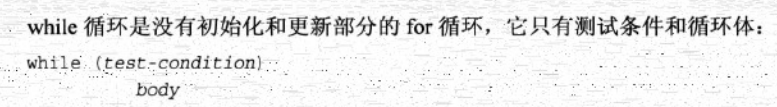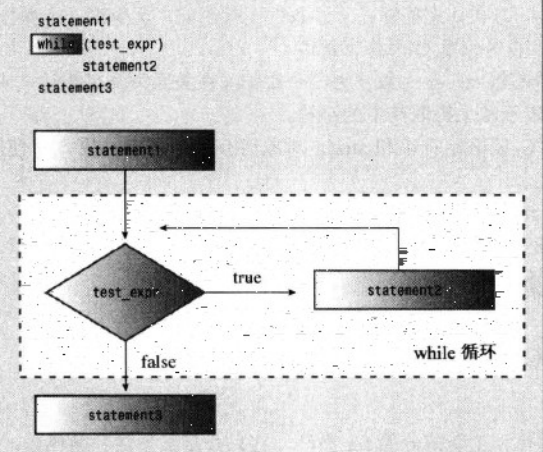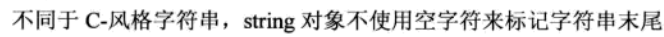## 示例1 遍历C风格字符串

#include <iostream>
const int Arsize = 20;
int main()
{
using namespace std;
char name[Arsize];
cin >> name;

int i = 0;
while(name[i])//等价于while(name[i] != '\0')，但前者更简洁也更常用
{
cout << name[i] << ": " << int (name[i]) << endl;//打印ASCII码必须强制转换
++i;
}
return 0;
}

Enter your name:
Manica
M: 77
a: 97
n: 110
i: 105
c: 99
a: 97


## 示例2 用clock()库函数创建延时循环

long wait = 0;
while(wait < 10000)
wait++;


ctime或者time.h中定义了一个符号常量：CLOCKS_PER_SEC,clock()返回的系统时间单位除以这个符号常量就得到了秒数。

#include <iostream>
#include <ctime>
int main()
{
using namespace std;
cout << "Enter the delay time, in seconds:";
float secs;
cin >> secs;
clock_t delay = secs * CLOCKS_PER_SEC;
clock_t start = clock();//获取当前系统时间
cout << "Starting...\a\n";
while(clock() - start < delay)
;
cout << "Done!\n";
return 0;
}

Enter the delay time, in seconds:5
Starting...
Done!


## 两种方式创建类型别名（#define typedef）

#define BYTE char//文件中所有BYTE会在编译前的预处理阶段背替换为char，BYTE是宏
typedef char BYTE;


#define byte_pointer char *
byte_pointer b1, b2;//预处理解文本替换后是char * b1, b2; 所以只有b1是char指针，b2只是char

typedef char * byte_pointer;//可以声明一系列变量
byte_pointer b1, b2;//二者都是char指针


# for和while循环本质上是相同的！但当循环次数不一定时常使用while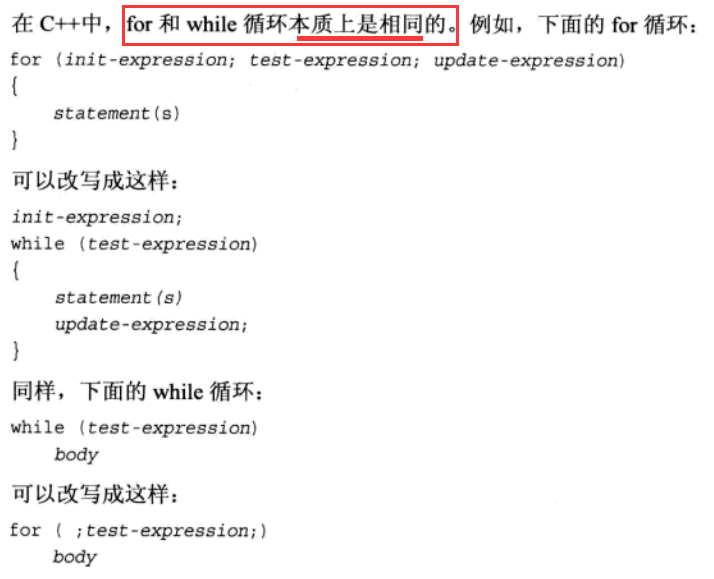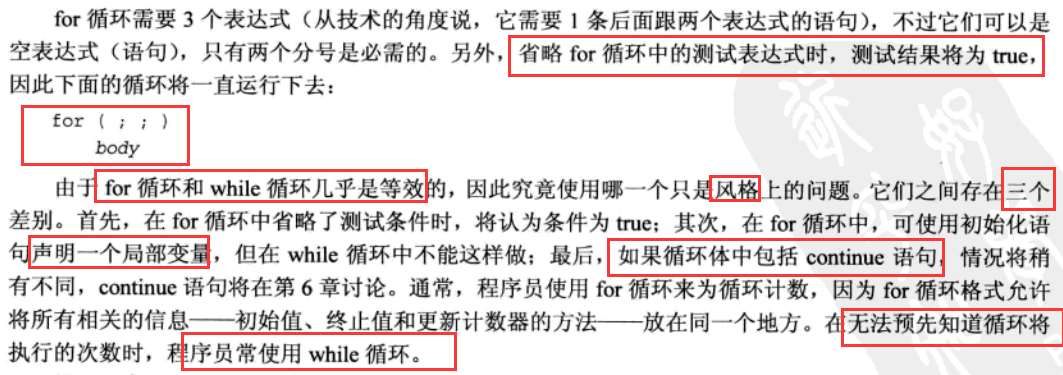## 一个奇葩示例

for

int i = 0;
for (;;i++)
{
if (30 <= i)
break;
}


while

int i = 0;
while(i < 30)
i++;


# do while循环(exit-condition loop：即先执行循环体再判断测试条件)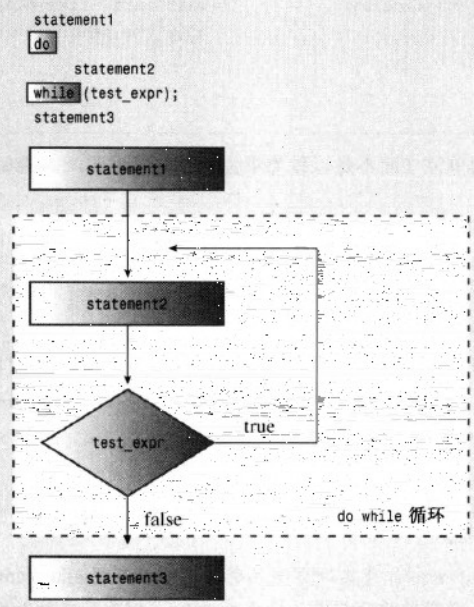## 示例1 猜数字

#include <iostream>
int main()
{
using std::cin;
using std::cout;

cout << "Enter an integer in the range 1-10 "
<< "to find my favorite number:\n";
int number;
do
{
cin >> number;
}while(number != 6);
cout << "Yes! 6 is my favorite number.\n";
return 0;
}

Enter an integer in the range 1-10 to find my favorite number:
2
8
3
9
10
4
6
Yes! 6 is my favorite number.


## do while比for循环更合理的例子

for

int i = 0;
for(;;) // a forever loop死循环
{
i++;
if(30 <= i)
break;
}


do while

int i = 0;
do
{
i++;
}while(i < 30);


07-3111
03-049006-2742
11-0463
04-212283
06-272万+
07-0841
10-1676
02-22122
06-20178